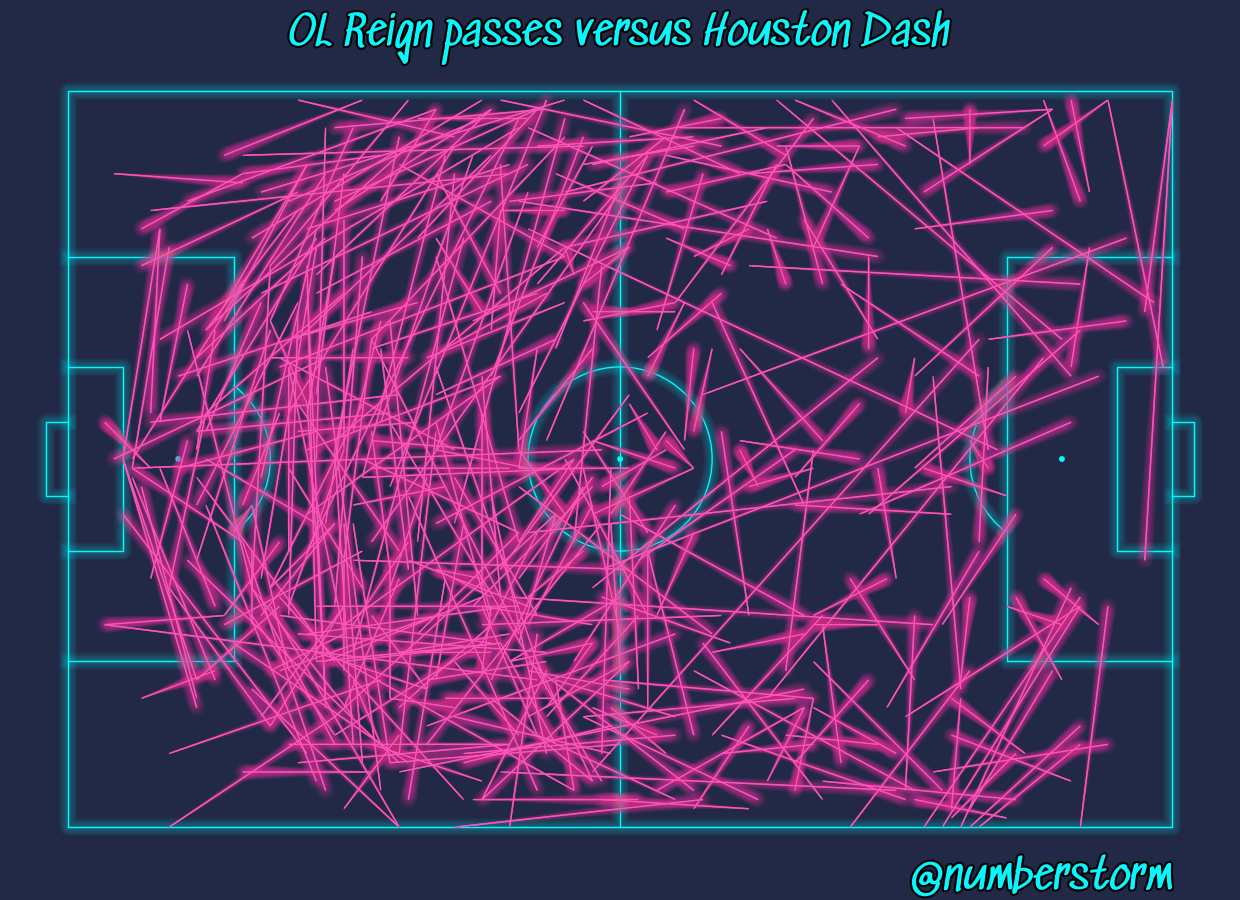# Cyberpunk theme

This example shows how to recreate the mplcyberpunk theme in mplsoccer.

It copies the technique of plotting the line once and adding glow effects. The glow effects are a loop of transparent lines increasing in linewidth so the center is more opaque than the outside.

```from mplsoccer import Pitch, FontManager, Sbopen
import matplotlib.patheffects as path_effects

parser = Sbopen()
df, related, freeze, tactics = parser.event(7478)

# get the team names
team1, team2 = df.team_name.unique()
# filter the dataset to completed passes for team 1
mask_team1 = (df.type_name == 'Pass') & (df.team_name == team1) & (df.outcome_name.isnull())
df_pass = df.loc[mask_team1, ['x', 'y', 'end_x', 'end_y', 'outcome_name']]

'SedgwickAve-Regular.ttf')
```

## Plotting cybperpunk passes

```LINEWIDTH = 1  # starting linewidth
DIFF_LINEWIDTH = 1.2  # amount the glow linewidth increases each loop
NUM_GLOW_LINES = 10  # the amount of loops, if you increase the glow will be wider

# in each loop, for the glow, we plot the alpha divided by the num_glow_lines
# I have a lower alpha_pass_line value as there is a slight overlap in
# the pass comet lines when using capstyle='round'
ALPHA_PITCH_LINE = 0.3
ALPHA_PASS_LINE = 0.15

# The colors are borrowed from mplcyberpunk. Try some of the following alternatives
# '#08F7FE' (teal/cyan), '#FE53BB' (pink), '#F5D300' (yellow),
# '#00ff41' (matrix green), 'r' (red), '#9467bd' (viloet)
BACKGROUND_COLOR = '#212946'
PASS_COLOR = '#FE53BB'
LINE_COLOR = '#08F7FE'

# plot as initial pitch and the lines with alpha=1
# I have used grid to get a title and endnote axis automatically, but you could you pitch.draw()
pitch = Pitch(line_color=LINE_COLOR, pitch_color=BACKGROUND_COLOR, linewidth=LINEWIDTH,
line_alpha=1, goal_alpha=1, goal_type='box')
fig, ax = pitch.grid(grid_height=0.9, title_height=0.06, axis=False,
endnote_height=0.04, title_space=0, endnote_space=0)
fig.set_facecolor(BACKGROUND_COLOR)
pitch.lines(df_pass.x, df_pass.y,
df_pass.end_x, df_pass.end_y,
capstyle='butt',  # cut-off the line at the end-location.
linewidth=LINEWIDTH, color=PASS_COLOR, comet=True, ax=ax['pitch'])

# plotting the titles and endnote
text_effects = [path_effects.Stroke(linewidth=3, foreground='black'),
path_effects.Normal()]
ax['title'].text(0.5, 0.3, f'{team1} passes versus {team2}',
path_effects=text_effects,
va='center', ha='center', color=LINE_COLOR, fontsize=30, fontproperties=fm.prop)
ax['endnote'].text(1, 0.5, '@numberstorm', va='center', path_effects=text_effects,
ha='right', color=LINE_COLOR, fontsize=30, fontproperties=fm.prop)

# plotting the glow effect. it is essentially a loop that plots the line with
# a low alpha (transparency) value and gradually increases the linewidth.
# This way the center will have more color than the outer area.
# you could break this up into two loops if you wanted the pitch lines to have wider glow
for i in range(1, NUM_GLOW_LINES + 1):
pitch = Pitch(line_color=LINE_COLOR, pitch_color=BACKGROUND_COLOR,
linewidth=LINEWIDTH + (DIFF_LINEWIDTH * i),
line_alpha=ALPHA_PITCH_LINE / NUM_GLOW_LINES,
goal_alpha=ALPHA_PITCH_LINE / NUM_GLOW_LINES,
goal_type='box')
pitch.draw(ax=ax['pitch'])  # we plot on-top of our previous axis from pitch.grid
pitch.lines(df_pass.x, df_pass.y,
df_pass.end_x, df_pass.end_y,
linewidth=LINEWIDTH + (DIFF_LINEWIDTH * i),
capstyle='round',  # capstyle round so the glow extends past the line
alpha=ALPHA_PASS_LINE / NUM_GLOW_LINES,
color=PASS_COLOR, comet=True, ax=ax['pitch'])
```Total running time of the script: ( 0 minutes 5.212 seconds)

Gallery generated by Sphinx-Gallery